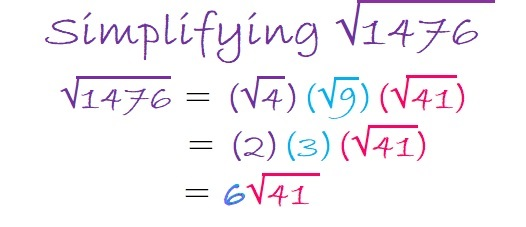# 1476 Mystery

### Today’s Puzzle:

To solve this mystery puzzle, first, gather some facts. I mean, common factors:
Common Factors of 6, 3, 12, and 9 are 1 and 3.
For 20 and 10 we have 2, 5, and 10.
And for 20 and 60, we can only use 5 or 10 because common factor, 20, is too big for a 1 to 12 puzzle.

Which common factors “Done it”? Don’t jump to conclusions. Remember, a good detective will use the facts and logic to figure out the mystery. Good luck!### Factors of 1476:

• 1476 is a composite number.
• Prime factorization: 1476 = 2 × 2 × 3 × 3 × 41, which can be written 1476 = 2² × 3² × 41
• 1476 has at least one exponent greater than 1 in its prime factorization so √1476 can be simplified. Taking the factor pair from the factor pair table below with the largest square number factor, we get √1476 = (√36)(√41) = 6√41
• The exponents in the prime factorization are 2, 2, and 1. Adding one to each exponent and multiplying we get (2 + 1)(2 + 1)(1 + 1) = 3 × 3 × 2 = 18. Therefore 1476 has exactly 18 factors.
• The factors of 1476 are outlined with their factor pair partners in the graphic below.Simplifying √1476 doesn’t have to be a mystery! Here’s one strategy to do it:### More Facts about the number 1476:

1476 is the sum of two squares:
30² + 24² = 1476

1476 is the hypotenuse of a Pythagorean triple:
324-1440-1476 which is 36 times (9-40-41),
and can also be calculated from 30² – 24², 2(30)(24), 30² + 24².